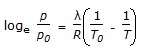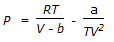# Chemical Engineering - Stoichiometry

### Exercise :: Stoichiometry - Section 7

1.

One kg of saturated steam at 100°C and 1.01325 bar is contained in a rigid walled vessel. It lias a volume of 1.673 m3. It cools to 98°C ; the saturation pressure is 0.943 bar ; one kg of water vapour under these conditions has a volume of 1.789 m3. The amount of water vapour condensed (in kg) is

 A. 0 B. 0.065 C. 0.1 D. 1

Explanation:

No answer description available for this question. Let us discuss.

2.

Which of the following holds good for a solution obeying Raoult's law (i.e., an ideal solution) (where, ΔH = heat of mixing, and ΔV = volume change on mixing ) ?

 A. ΔH = 1 (+ ve)and Δ V = -ve B. ΔH = 0 C. ΔV = 0 D. both (b) and (c)

Explanation:

No answer description available for this question. Let us discuss.

3.

Which equation is not an equation of state ?

 A. PV = RT + B/V + y/V2 + .... B. (P + a/V2)(V-b) = RT C.D.Explanation:

No answer description available for this question. Let us discuss.

4.

Number of gm moles of solute dissolved in 1 kg of solvent is called its

 A. normality B. molarity C. molality D. formality

Explanation:

No answer description available for this question. Let us discuss.

5.

Size range of the colloidals particles is

 A. 5 - 200 milli-microns B. 50 - 200 microns C. 500 - 1000 microns D. 10 - 50 Angstrom